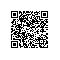# https://yq.aliyun.com/articles/85369# ◆ ◆ ◆

#### 使用可重用的保留数据法来避免在交互式数据分析中出现过拟合from numpy import *

def Thresholdout(sample, holdout, q):

# function q is what you’re “testing” – e.g., model loss

sample_mean = mean([q(x)  for x in sample])

holdout_mean = mean([q(x)  for x in holdout])

sigma = 1.0 / sqrt(len(sample))

threshold = 3.0*sigma

if (abs(sample_mean – holdout_mean)

< random.normal(threshold, sigma) ):

# q does not overfit: your “training estimate” is good

return sample_mean

else:

# q overfits (you may have overfit using your training data)

return holdout_mean + random.normal(0, sigma)

# ◆ ◆ ◆

#### 通过局部近似来解释你的黑盒模型

原文发布时间为：2016-09-11使用钉钉扫一扫加入圈子
+ 订阅